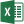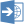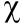Scale-up Suite Help
Export fitting results
Fitting reports

A Fitting report can be generated in Excel or HTML format usingandfrom the Fitting toolbar. These reports include general run information, final parameter values and statistics of the fit (confidence intervals, covariance matrix etc.) and the algorithm and solver options used.

To export fitting results to Excel click on the Excel report iconin the Fitting toolbar.  Alternatively, to view the results before exporting, click on the Preview Statisticsicon.

The Fitting Report includes the following information:

• The date, time, model name, user name
• Parameter Inputs: initial parameter values, confidence level (90%, 95%, 99%), parameter constraints (min and max values), parameter units
• Parameter Outputs: final ‘free’ parameter values, final constrained parameter values, confidence intervals on ‘free’ parameters, variance-covariance matrix for ‘free’ parameters, correlation matrix, standard errors on ‘free’ parameters, t statistic for ‘free parameters’, critical t statistic for free parameters, probability from a t distribution based on the t statistic for the parameter
• Data Inputs: the Scenarios selected and the data series selected (in each selected Scenario)
• Data Outputs: number of data points per series, number of series, number of scenarios
• Degree of fit Outputs: degrees of freedom, sum of squares (SSQ) for each series, r-squared and coefficient of determination for each series
• Model and residual outputs: Final SSQ or chi-squared, log likelihood, Goodness of fit (Q), average error value, F statistic, critical F statistic and probability from the F distribution, model selection criterion, mean absolute percentage deviation of residuals, autocorrelation of residuals, normalized skewness and kurtosis of residuals
• Algorithm & Solver Inputs: solver selection, solver accuracy, algorithm selection, tolerance, number of restarts
• Algorithm & solver Outputs: number of fitting iterations, number of solver runs, time per solver run, simulation time
• Table of residuals (all measured and predicted values compared) and the assumed standard deviation of the error distribution for each datapoint
• Covariance matrix and correlation matrix of model residuals
• A table of lack of fit (SSQ or chi-squared) values and estimated parameter values versus fitting iteration.